# 第三次作业

#include<stdio.h>
int main()
{
int i,sum;
sum=0;
for(i=1;i<=100;i++){
sum=sum+i;
}
printf("sum = %d",sum);
return 0;
}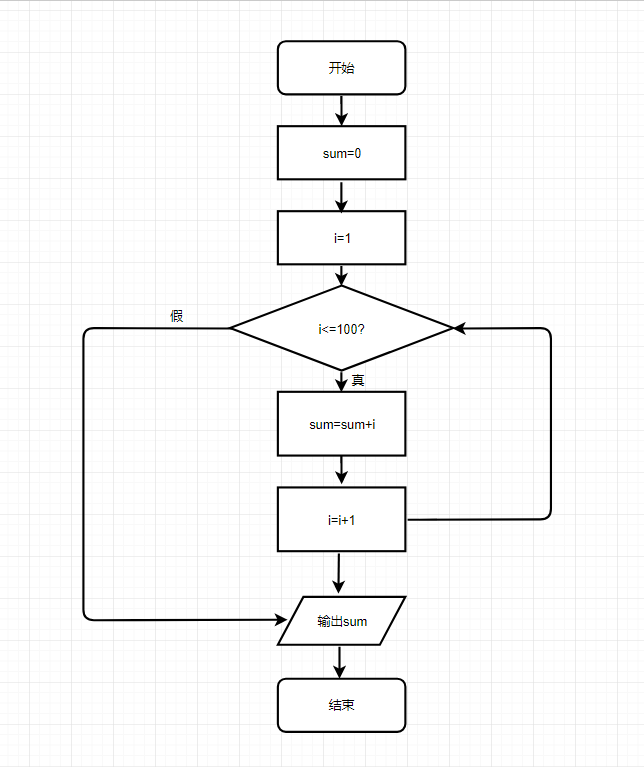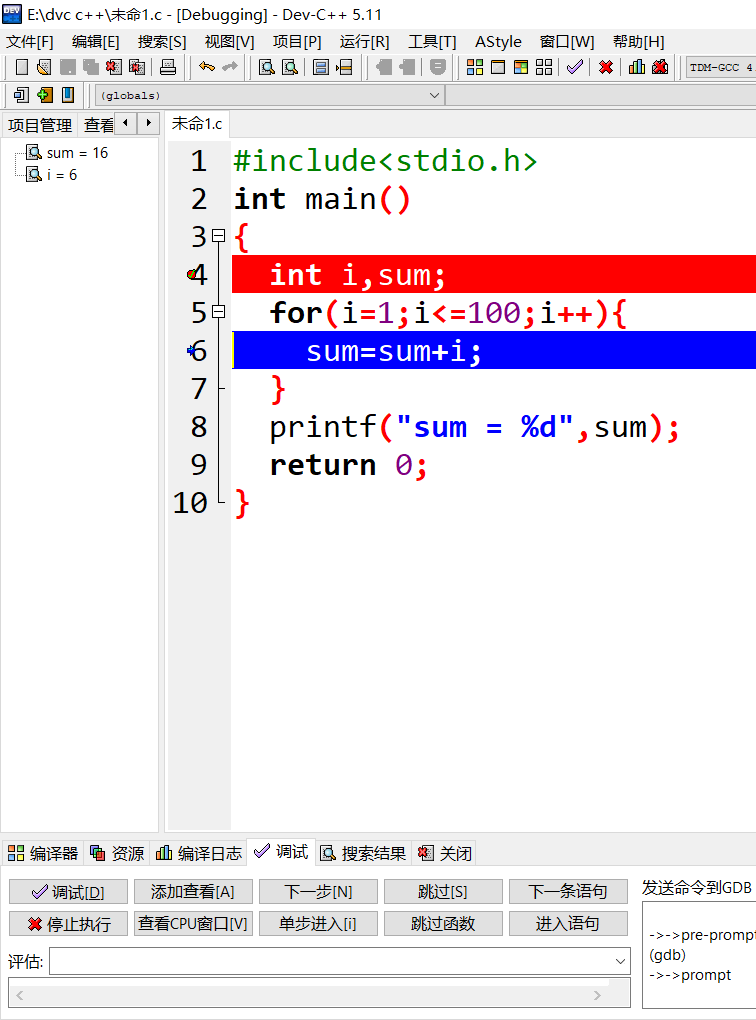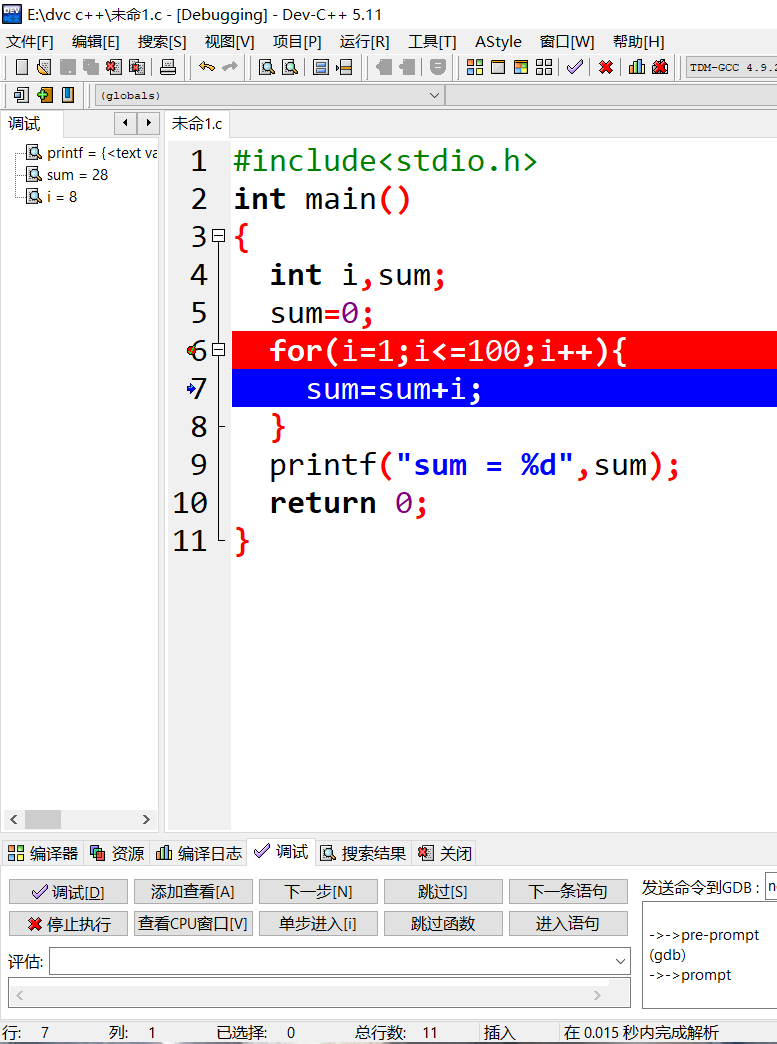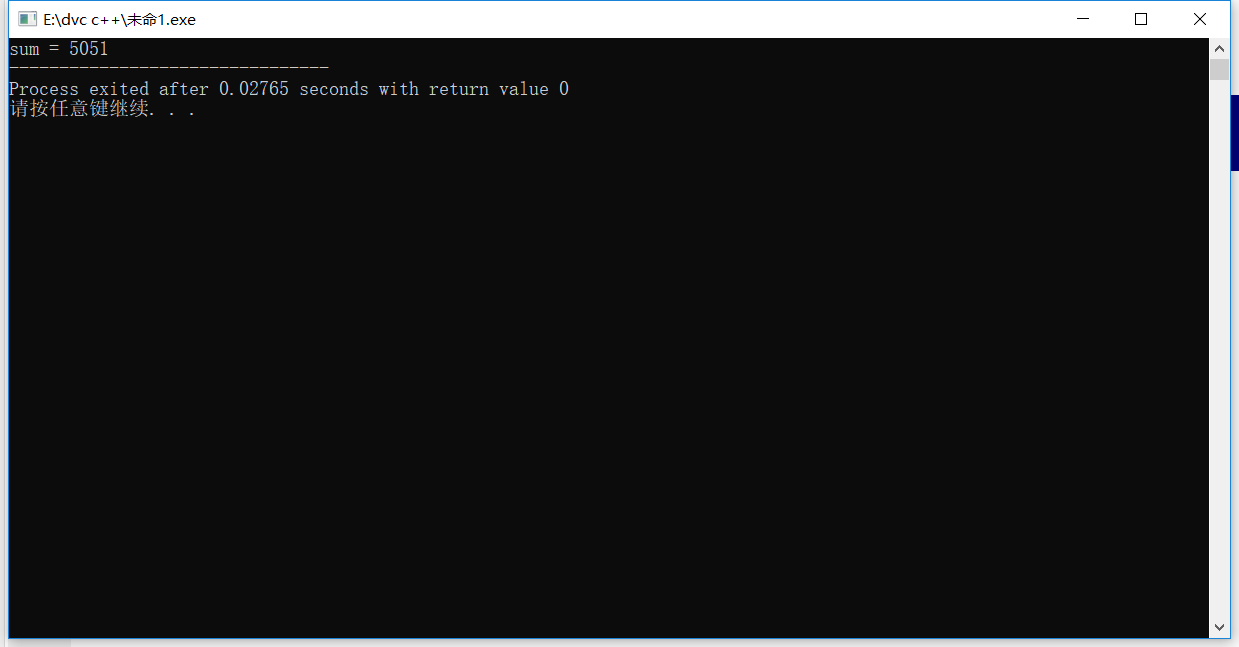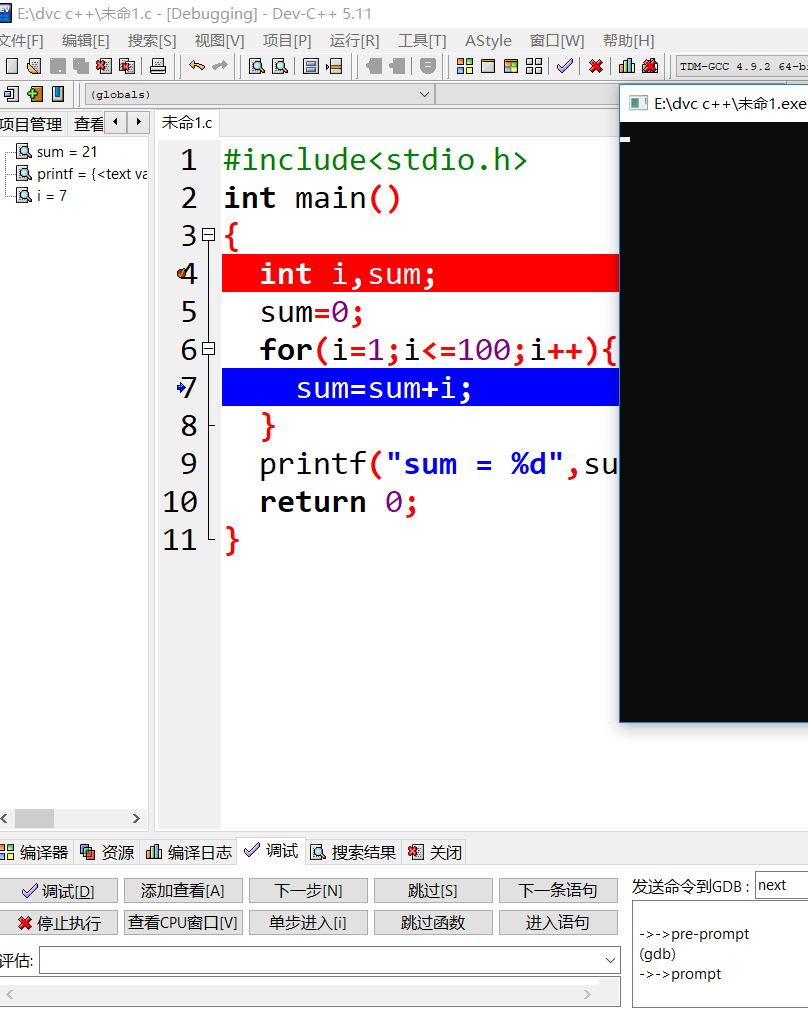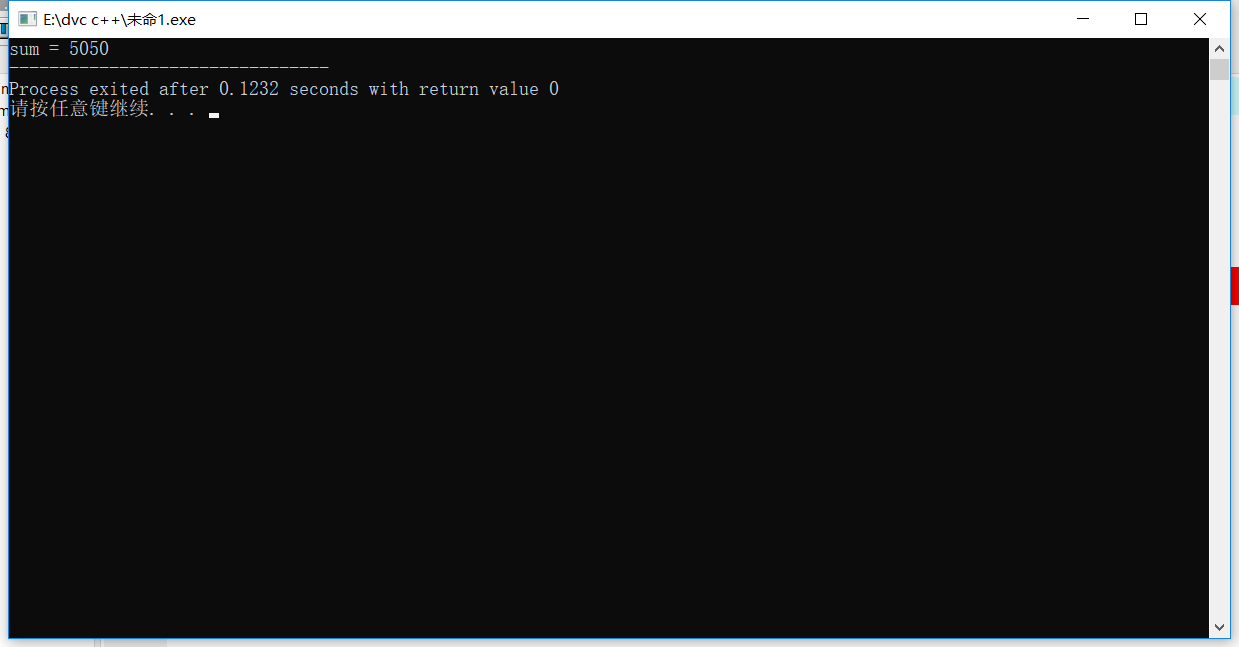个人总结和遇到的问题

#include<stdio.h>
int main()
{
int a=0,i=2000;
scanf("%d",&a);
if(a<i){
printf("Invalid year!");
}
else{
while(i<=a){
if(a>2100){
printf("Invalid year!");
break;
}
else if(a>=2004){
i++;
if(i%100!=0){
if(i%4==0){
printf("%d\n",i);
}
}
else if(i%400==0){
printf("%d\n",i);
}
}
else{
printf("None");
break;
}
}}
return 0;
}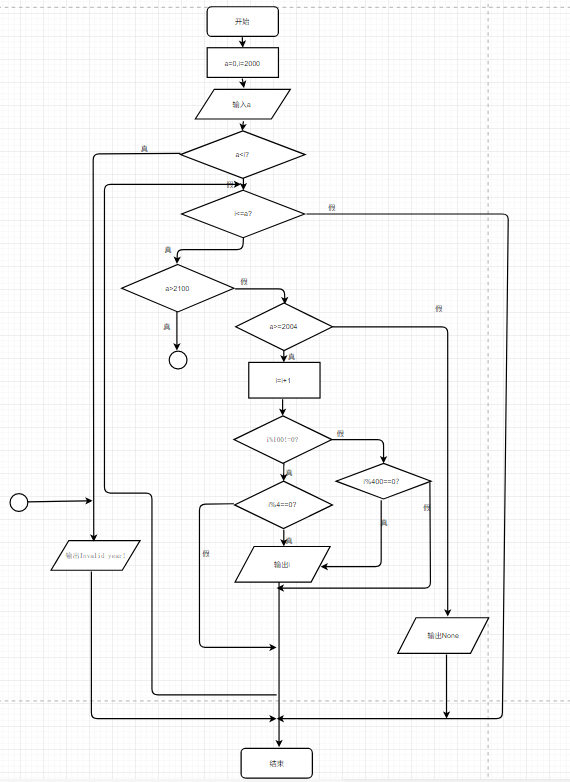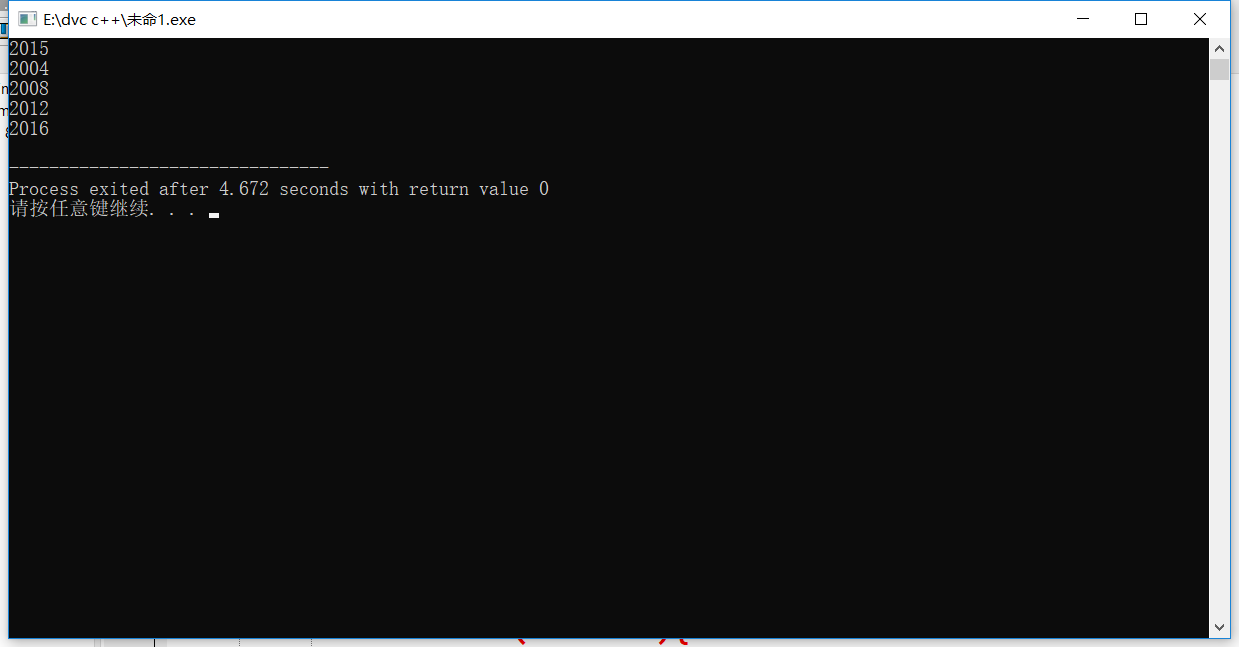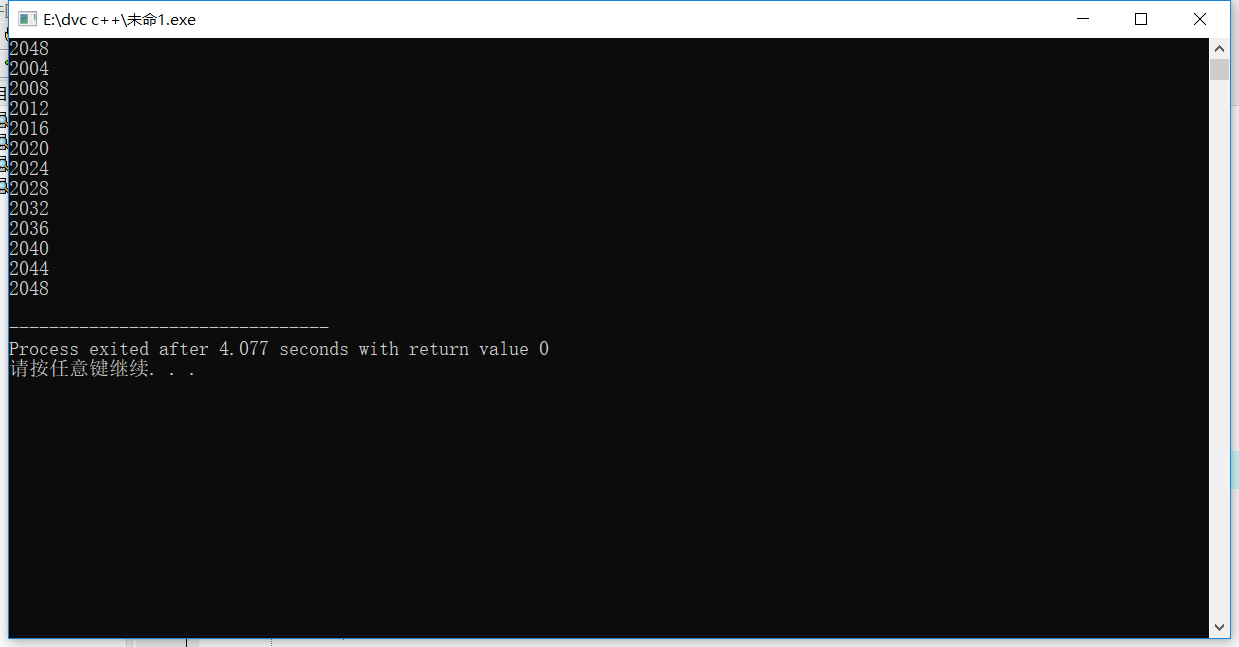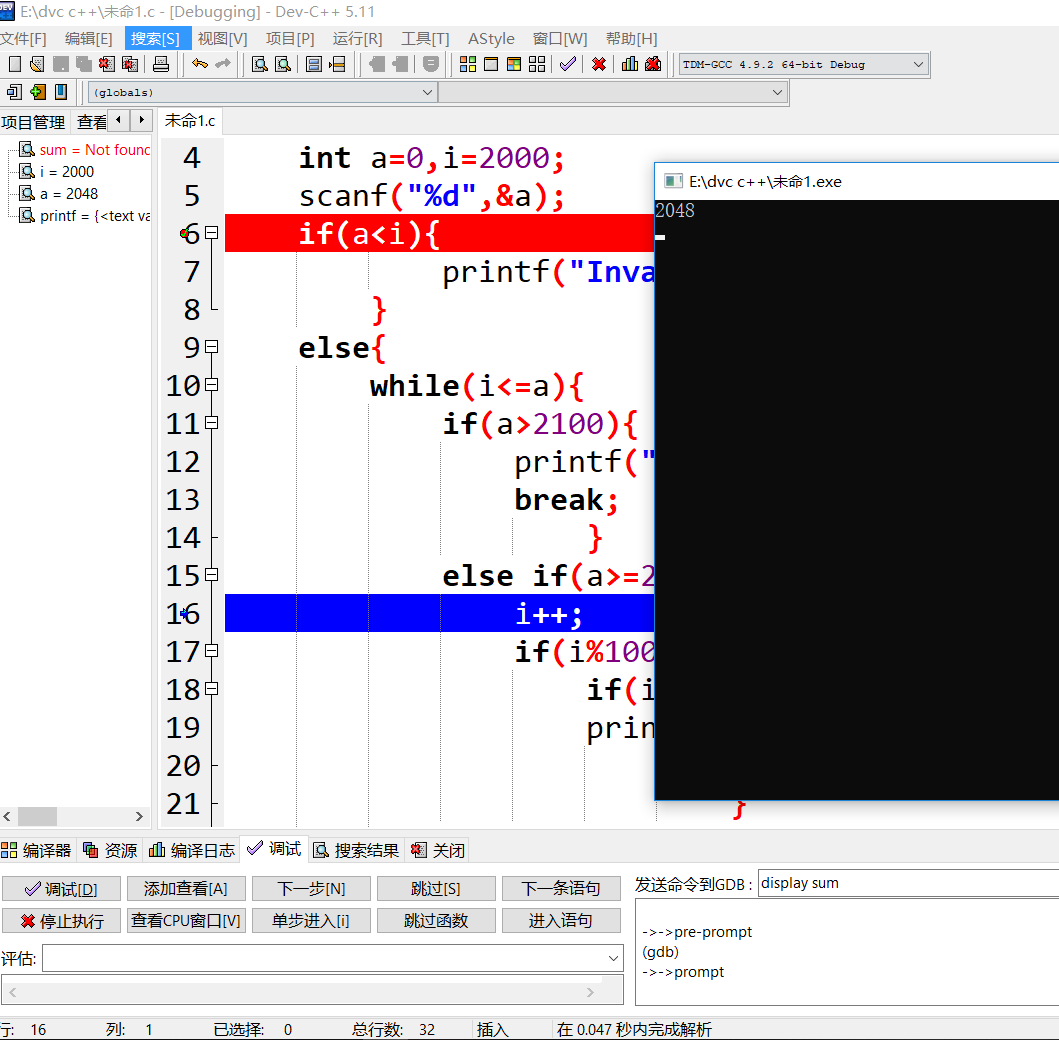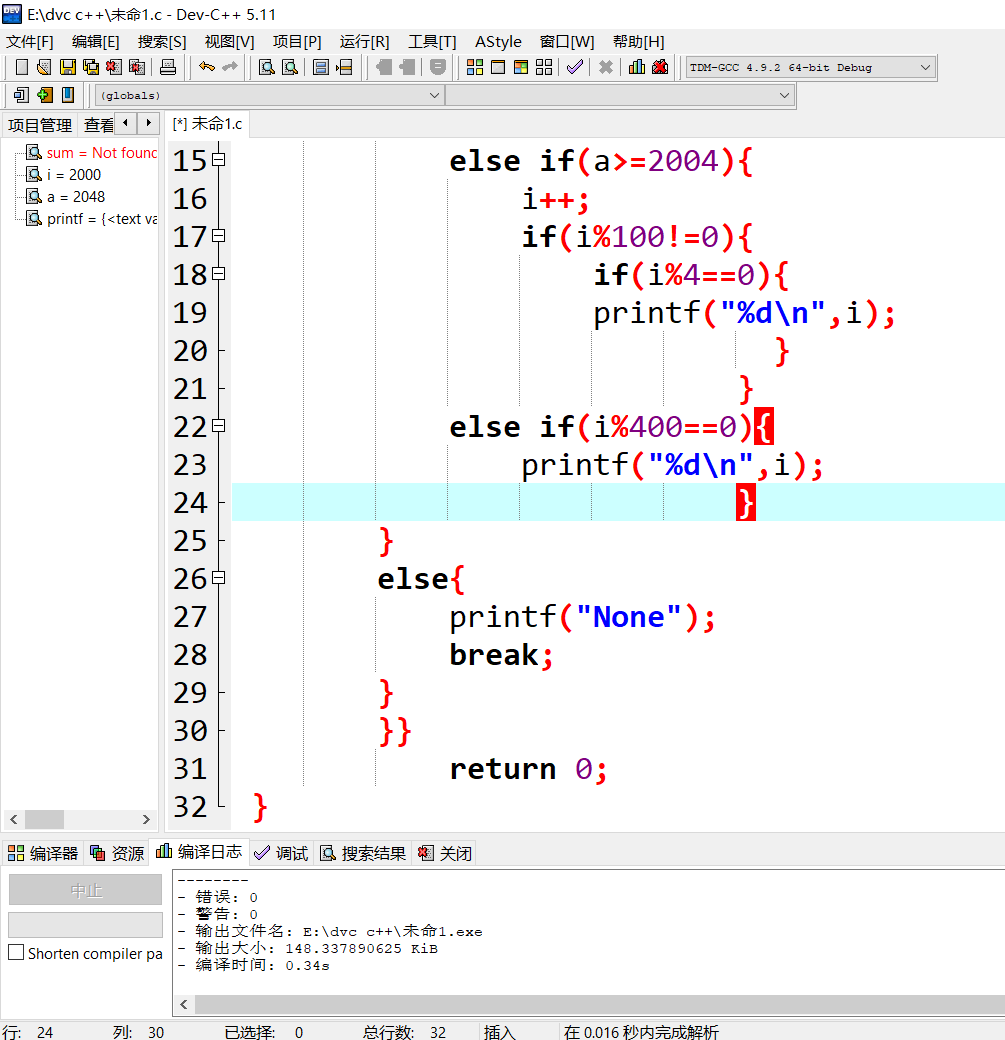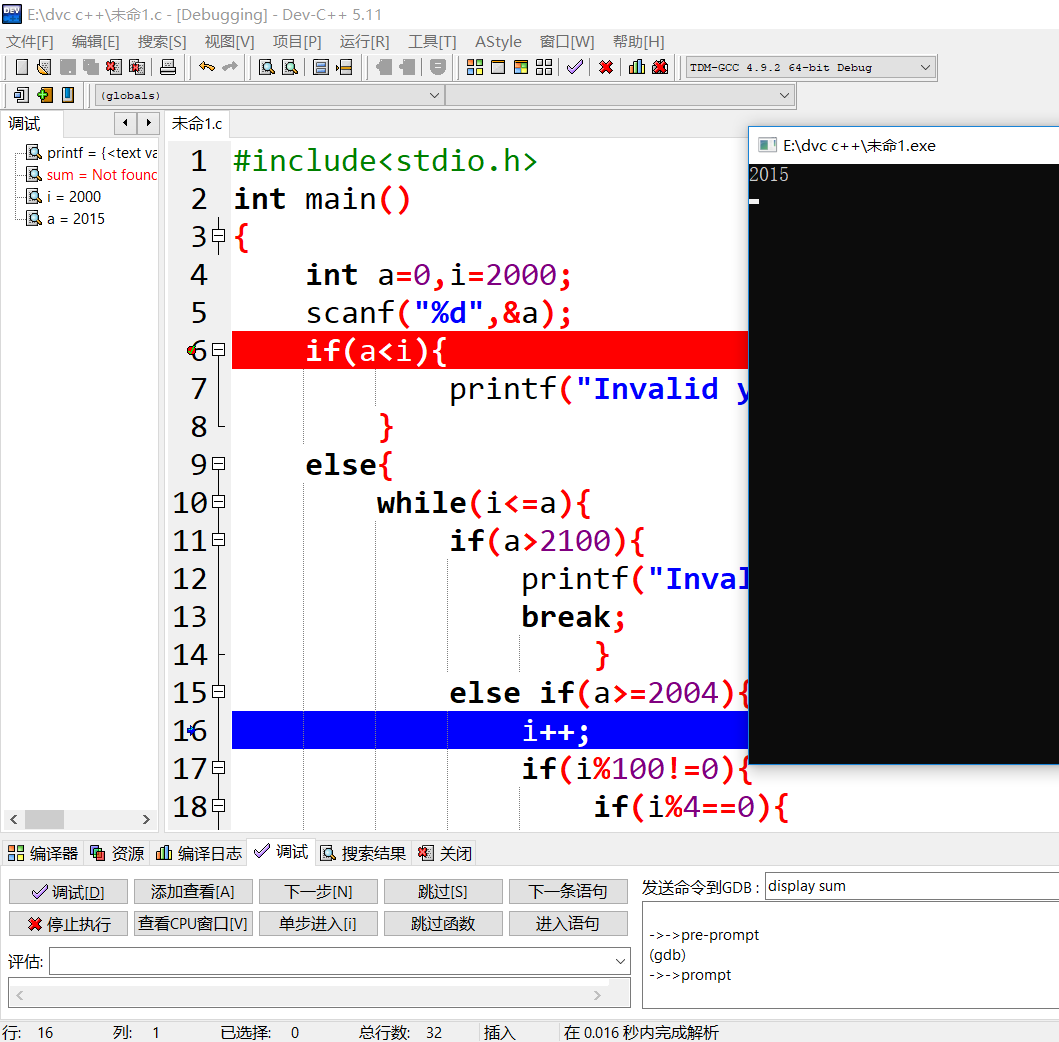个人总结和遇到的问题

#include<stdio.h>
int main()
{
int a,b,i,n,count=0,sum=0;
int flag=1;
scanf("%d %d",&a,&b);
for(i=a;i<=b;i++){
for(n=2;n<i;n++){
flag=1;
if(i%n==0){
flag=0;
break;
}
}
if(flag==1&&i!=1){
count++;
sum=sum+i;}
}
printf("%d %d",count,sum);
return 0;
}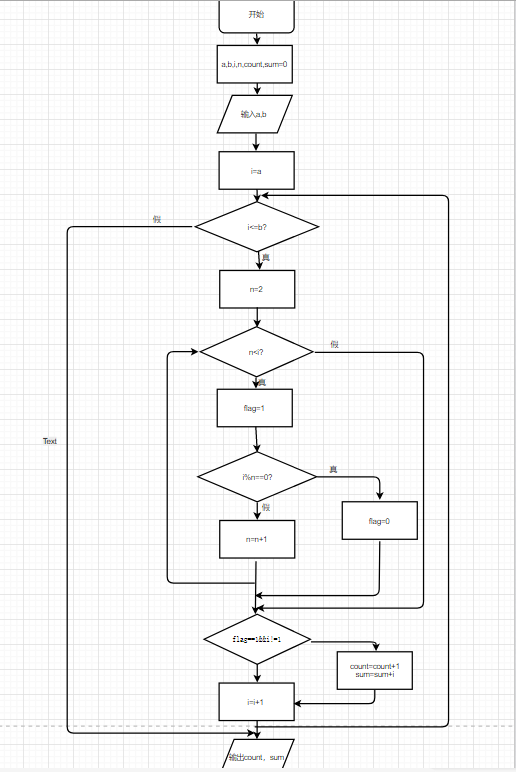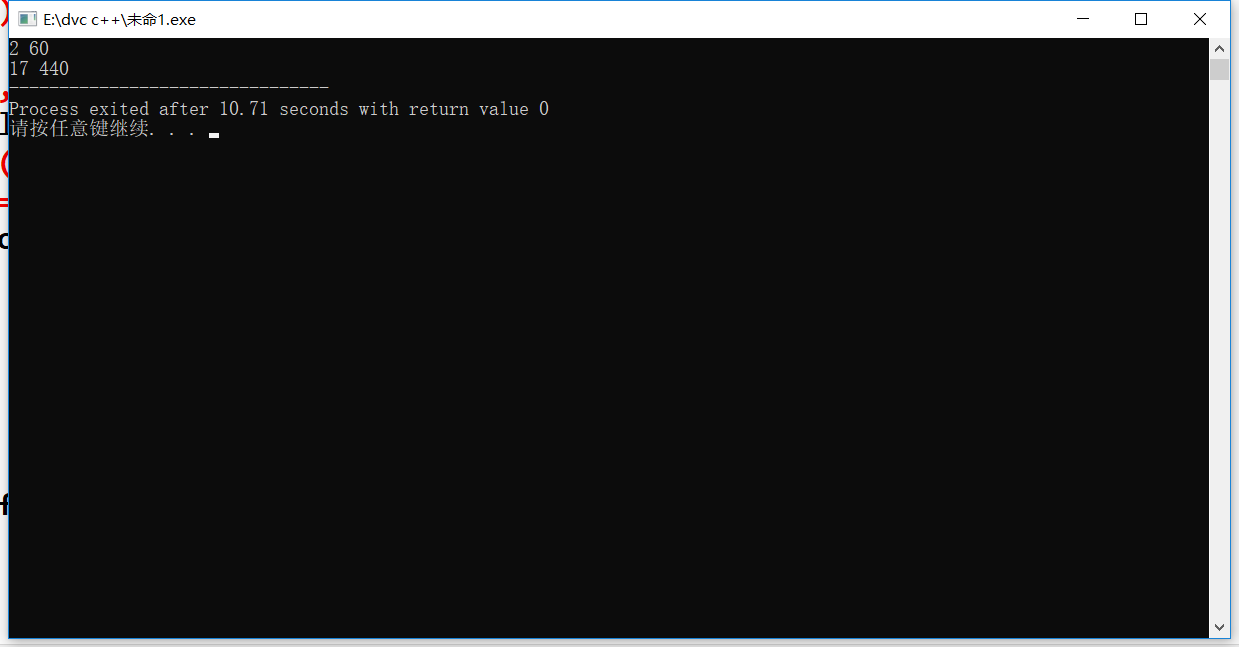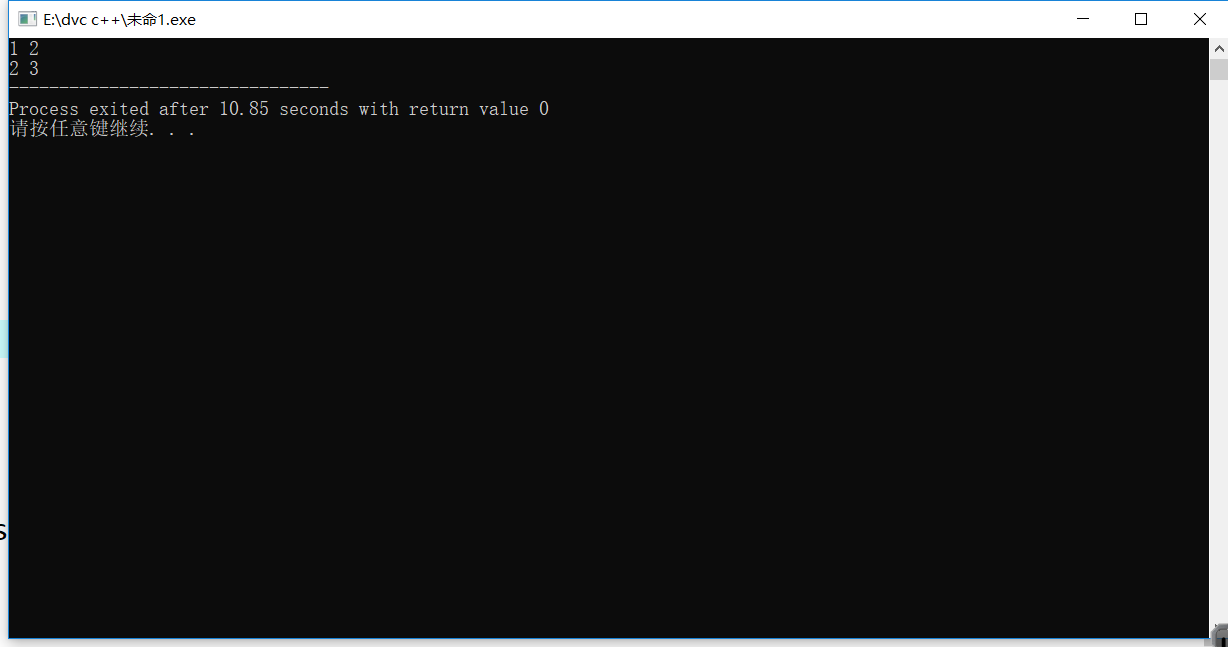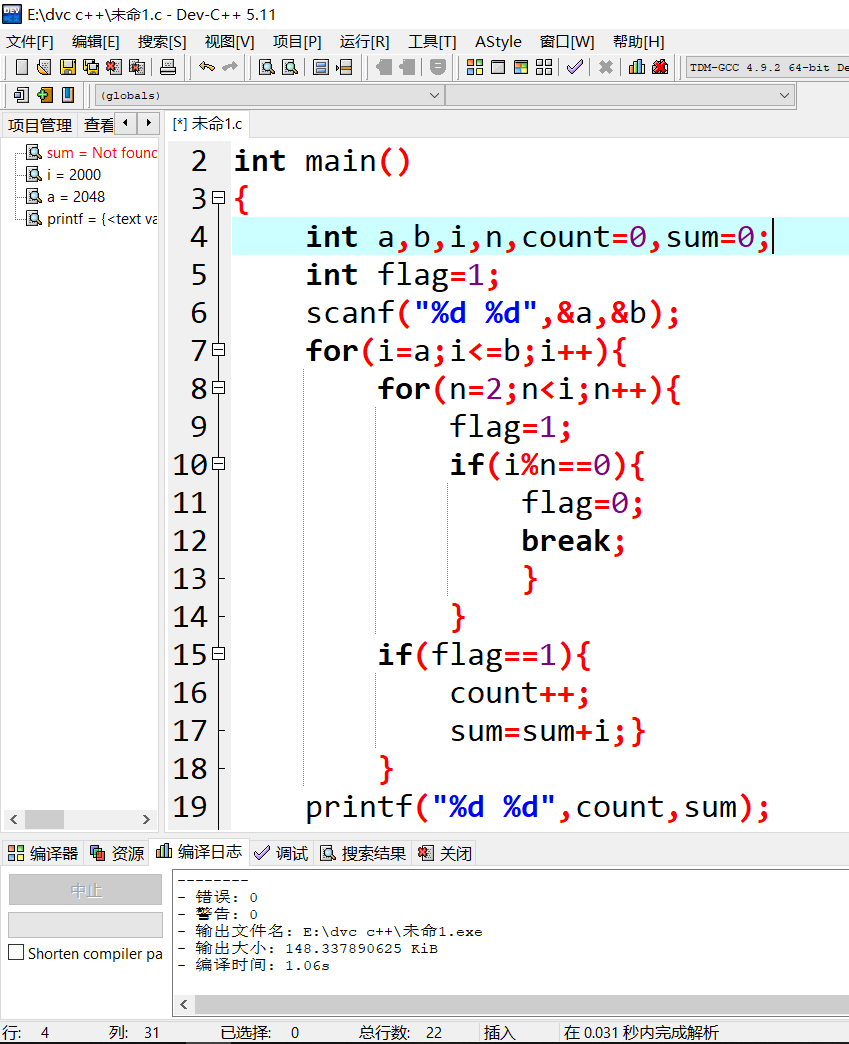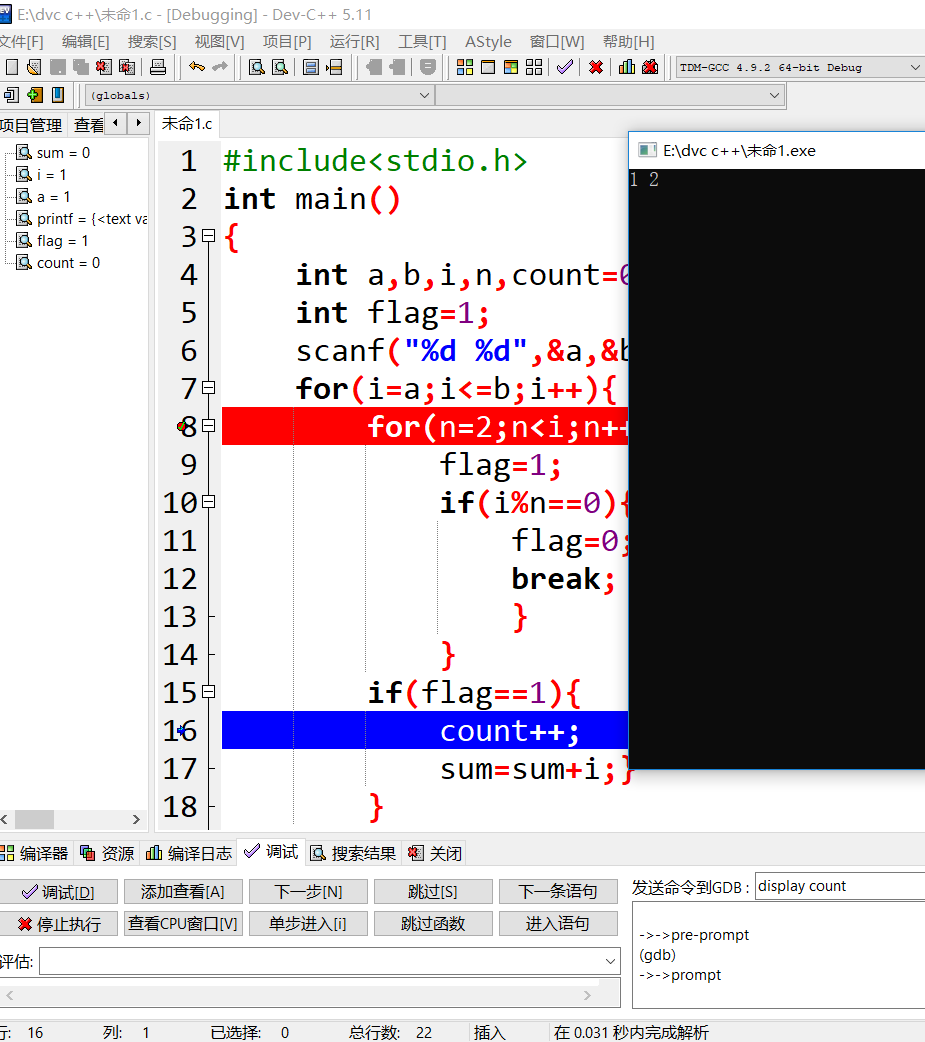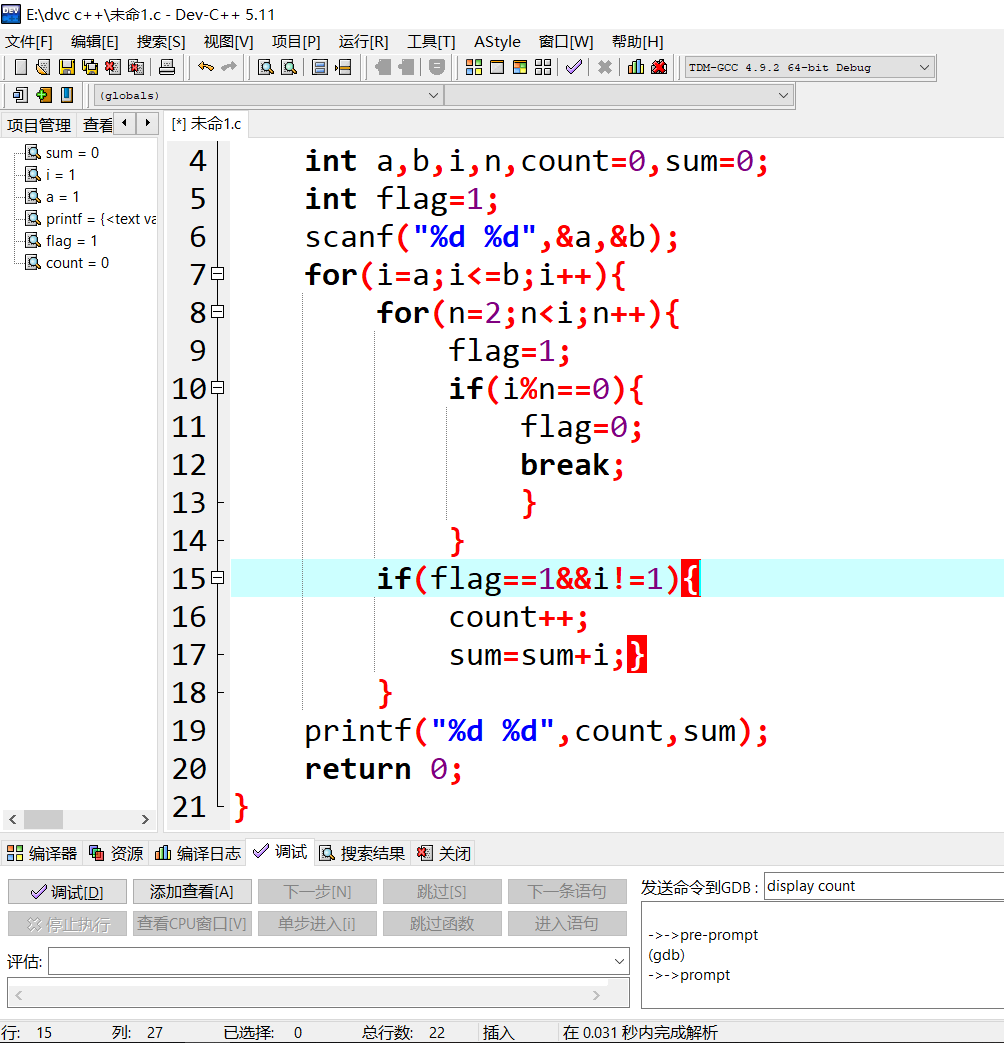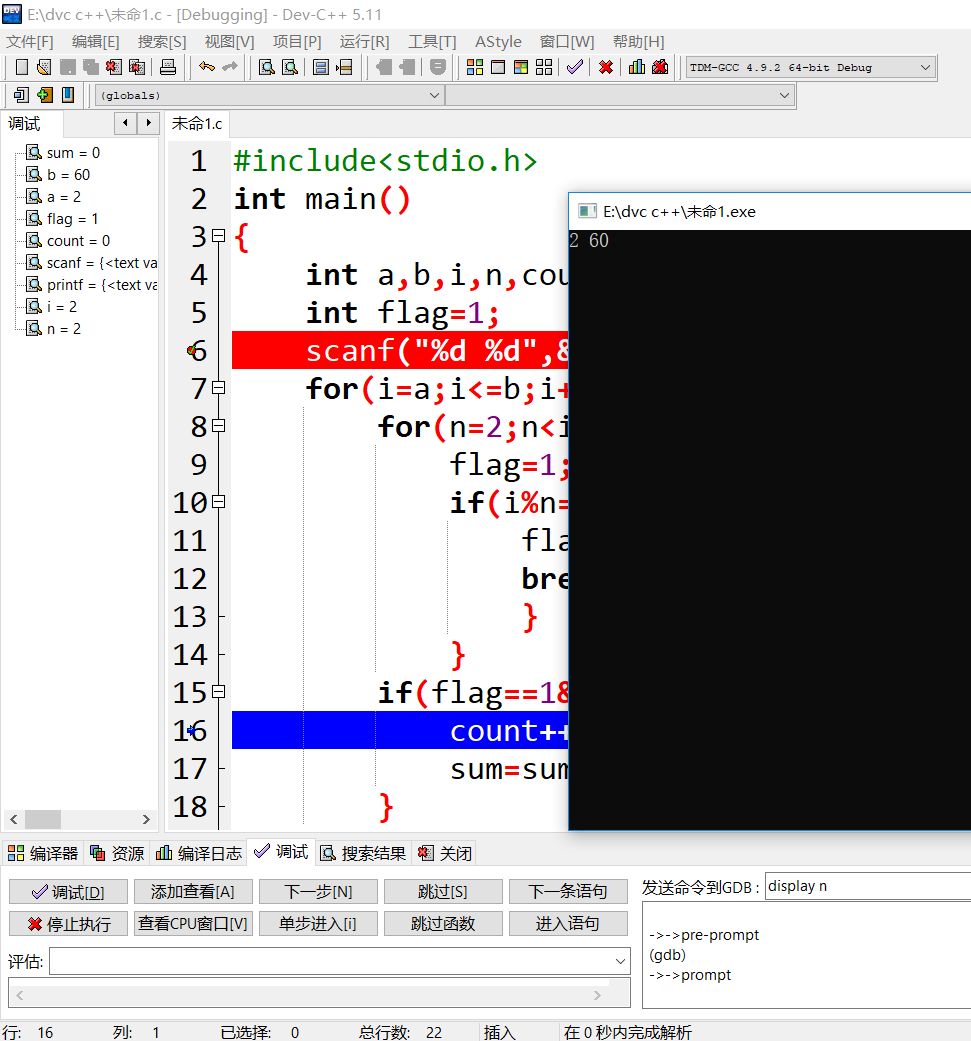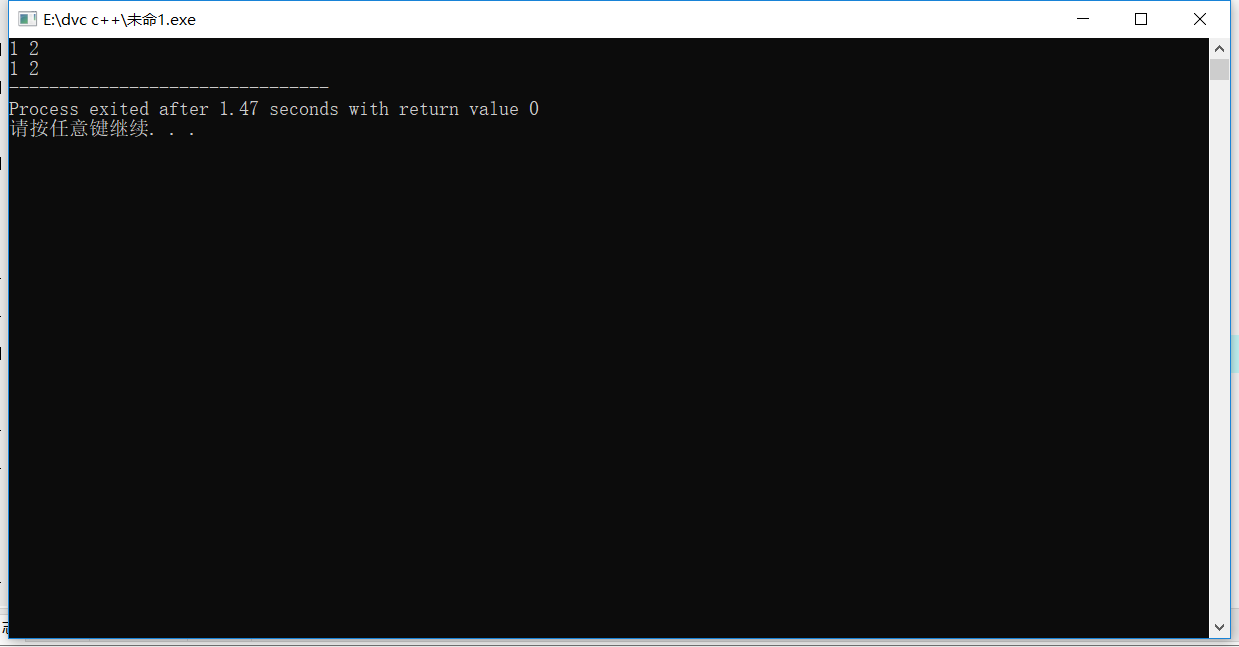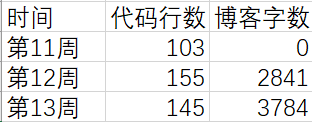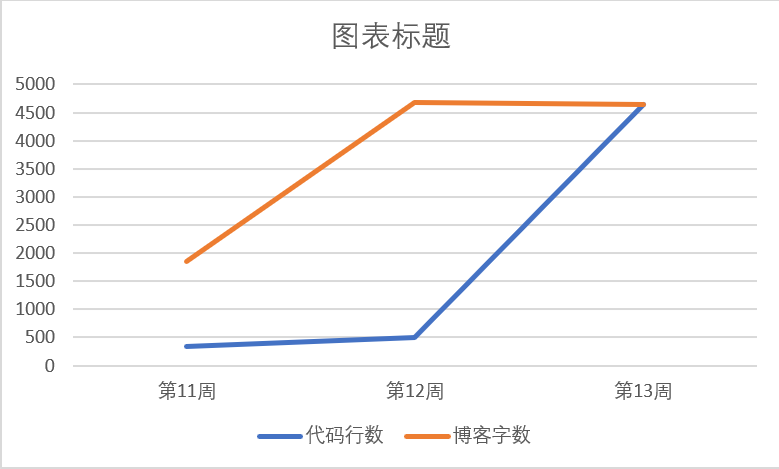posted @ 2018-11-27 19:35  张春宇  阅读(201)  评论(12编辑  收藏  举报